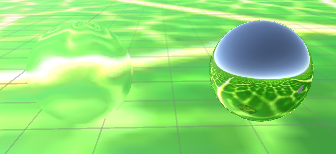# Making a texture warp using geometry with shaders

I have a shader that when applied to an object, will render everything behind that object to a texture and then warp it using a bumpmap. The result is something that looks like light refraction but isn’t.The shader I’m talking about is applied to the sphere on the left. To the right is a sphere using the standard shader with it’s smoothness and metallic turned up to 1.0.

The sphere on the right is using a cubemap from a reflection probe that is higher up in the scene, and it’s warping the image spherically, because it’s a sphere. this makes sense. The sphere on the left doesn’t really make sense because it’s warping its texture with this bumpmap

I was hoping someone could help me find a way to warp the image the way the standard shader warps its cubemap instead of using a bumpmap. I would appreciate it, and thanks in advance.

oh, here’s the actual code for the shader… that might be useful

``````Shader "Custom/GlassShader"
{
Properties
{
_MainTex ("Base (RGB) Trans (A)", 2D) = "white" {}
_Colour ("Colour", Color) = (1,1,1,1)

_BumpMap ("Noise text", 2D) = "bump" {}
_Magnitude ("Magnitude", Range(0,1)) = 0.05
}

{
Tags {"Queue"="Transparent" "IgnoreProjector"="True" "RenderType"="Opaque"}
ZWrite On Lighting Off Cull Off Fog { Mode Off } Blend One Zero

GrabPass { "_GrabTexture" }

Pass
{
CGPROGRAM
#pragma vertex vert
#pragma fragment frag
#include "UnityCG.cginc"

sampler2D _GrabTexture;

sampler2D _MainTex;
fixed4 _Colour;

sampler2D _BumpMap;
float  _Magnitude;

struct vin_vct
{
float4 vertex : POSITION;
float4 color : COLOR;
float2 texcoord : TEXCOORD0;
};

struct v2f_vct
{
float4 vertex : POSITION;
fixed4 color : COLOR;
float2 texcoord : TEXCOORD0;

float4 uvgrab : TEXCOORD1;
};

// Vertex function
v2f_vct vert (vin_vct v)
{
v2f_vct o;
o.vertex = mul(UNITY_MATRIX_MVP, v.vertex);
o.color = v.color;

o.texcoord = v.texcoord;

o.uvgrab = ComputeGrabScreenPos(o.vertex);
return o;
}

// Fragment function
half4 frag (v2f_vct i) : COLOR
{
half4 mainColour = tex2D(_MainTex, i.texcoord);

half4 bump = tex2D(_BumpMap, i.texcoord);
half2 distortion = UnpackNormal(bump).rg;

i.uvgrab.xy += distortion * _Magnitude;

fixed4 col = tex2Dproj( _GrabTexture, UNITY_PROJ_COORD(i.uvgrab));
return col * mainColour * _Colour;
}

ENDCG
}
}
}
``````

The first one is generally how you render refraction in realtime engines. Actually calculating refraction is way too intensive for most systems. As for the standard shader, it doesn’t really ‘distort’ the texture, but more use realtime texture coordinates based off the reflection vector from the camera to the surface normal (how real reflection works). The Unity Manual explains this, and has multiple examples that use reflection mapping with different techniques.

The following is the example from the Unity Manual that calculates reflection based off the local reflection probe (does not include normal mapping, but that is also in the manual).

``````Shader "Unlit/SkyReflection"
{
{
Pass
{
CGPROGRAM
#pragma vertex vert
#pragma fragment frag
#include "UnityCG.cginc"

struct v2f {
half3 worldRefl : TEXCOORD0;
float4 pos : SV_POSITION;
};

v2f vert (float4 vertex : POSITION, float3 normal : NORMAL)
{
v2f o;
o.pos = UnityObjectToClipPos(vertex);
// compute world space position of the vertex
float3 worldPos = mul(_Object2World, vertex).xyz;
// compute world space view direction
float3 worldViewDir = normalize(UnityWorldSpaceViewDir(worldPos));
// world space normal
float3 worldNormal = UnityObjectToWorldNormal(normal);
// world space reflection vector
o.worldRefl = reflect(-worldViewDir, worldNormal);
return o;
}

fixed4 frag (v2f i) : SV_Target
{
// sample the default reflection cubemap, using the reflection vector
half4 skyData = UNITY_SAMPLE_TEXCUBE(unity_SpecCube0, i.worldRefl);
// decode cubemap data into actual color
half3 skyColor = DecodeHDR (skyData, unity_SpecCube0_HDR);
// output it!
fixed4 c = 0;
c.rgb = skyColor;
return c;
}
ENDCG
}
}
}
``````